Miscellaneous

Chapter 15 Class 11 Statistics
Serial order wise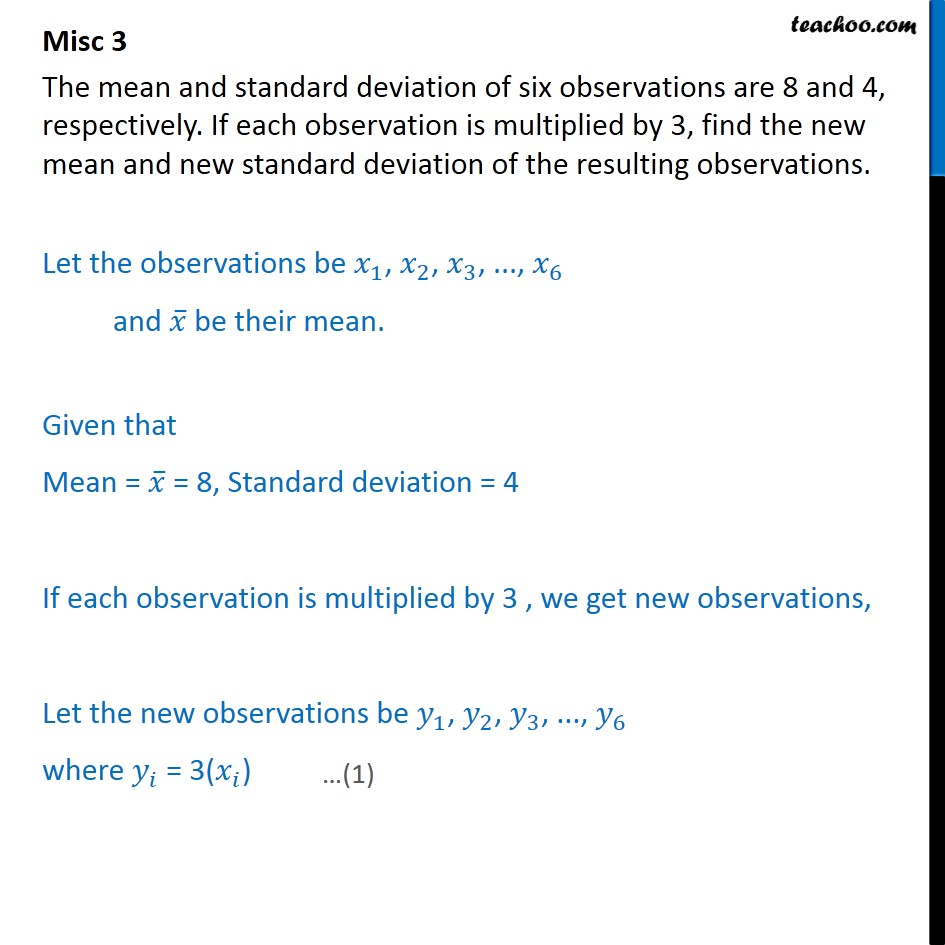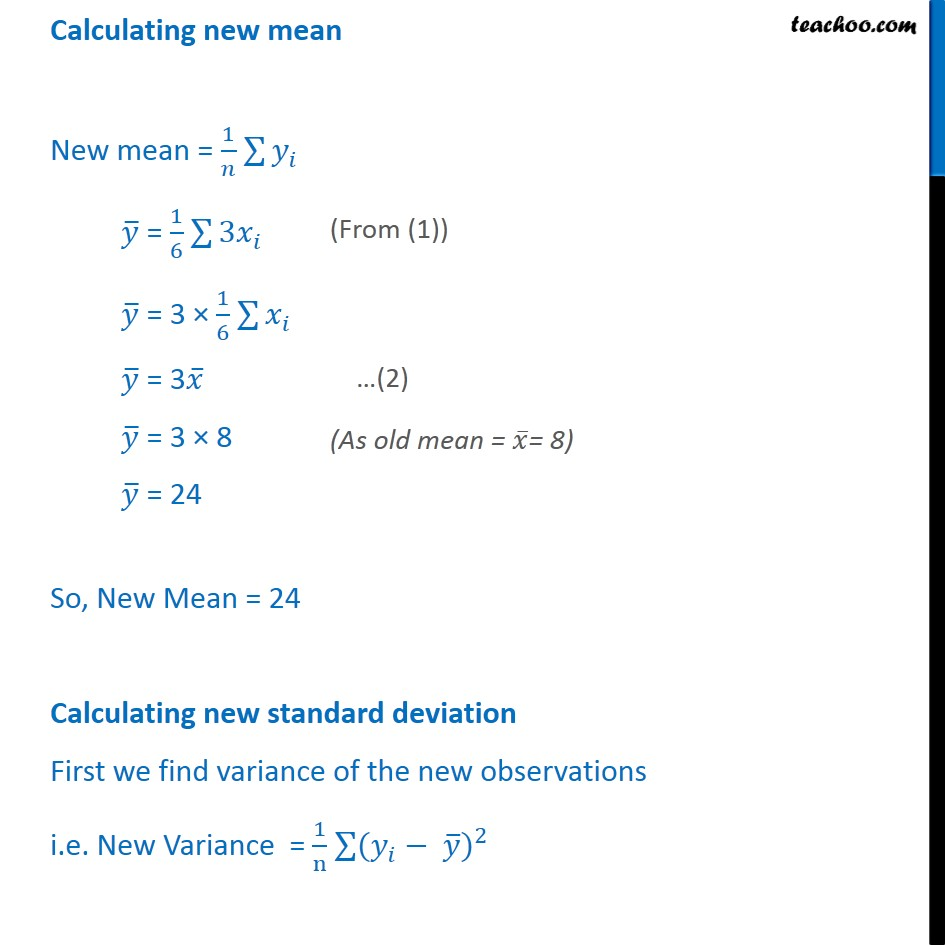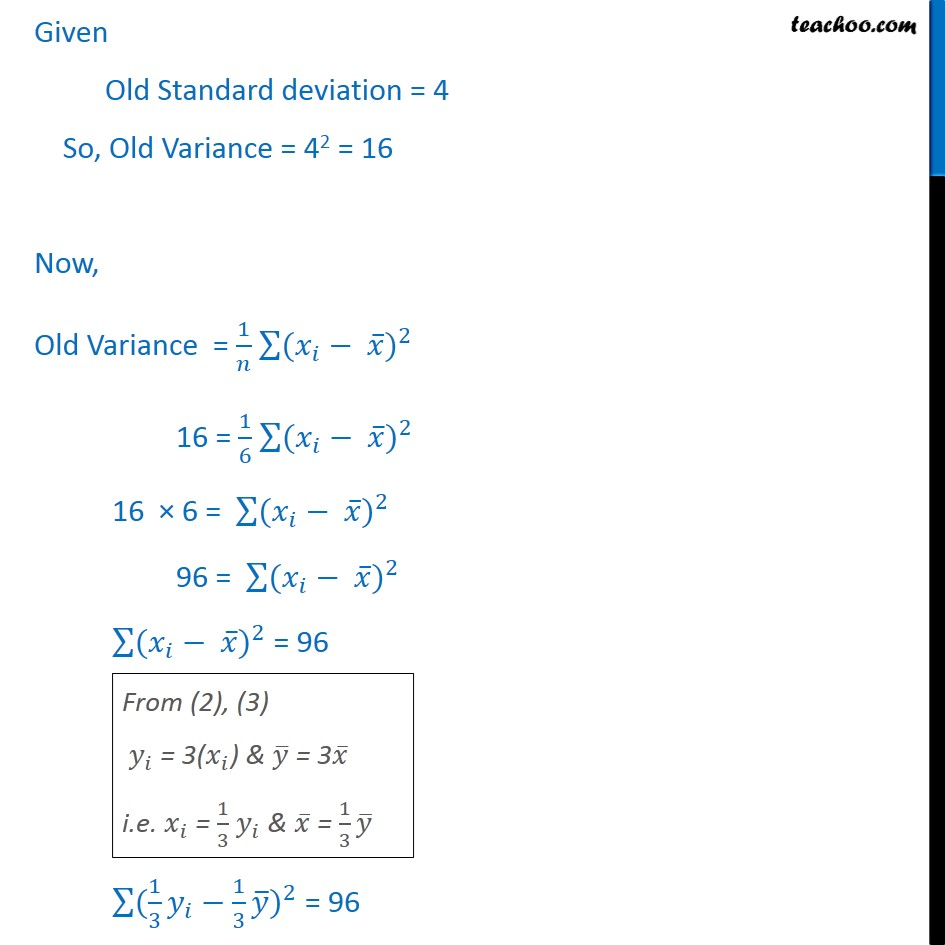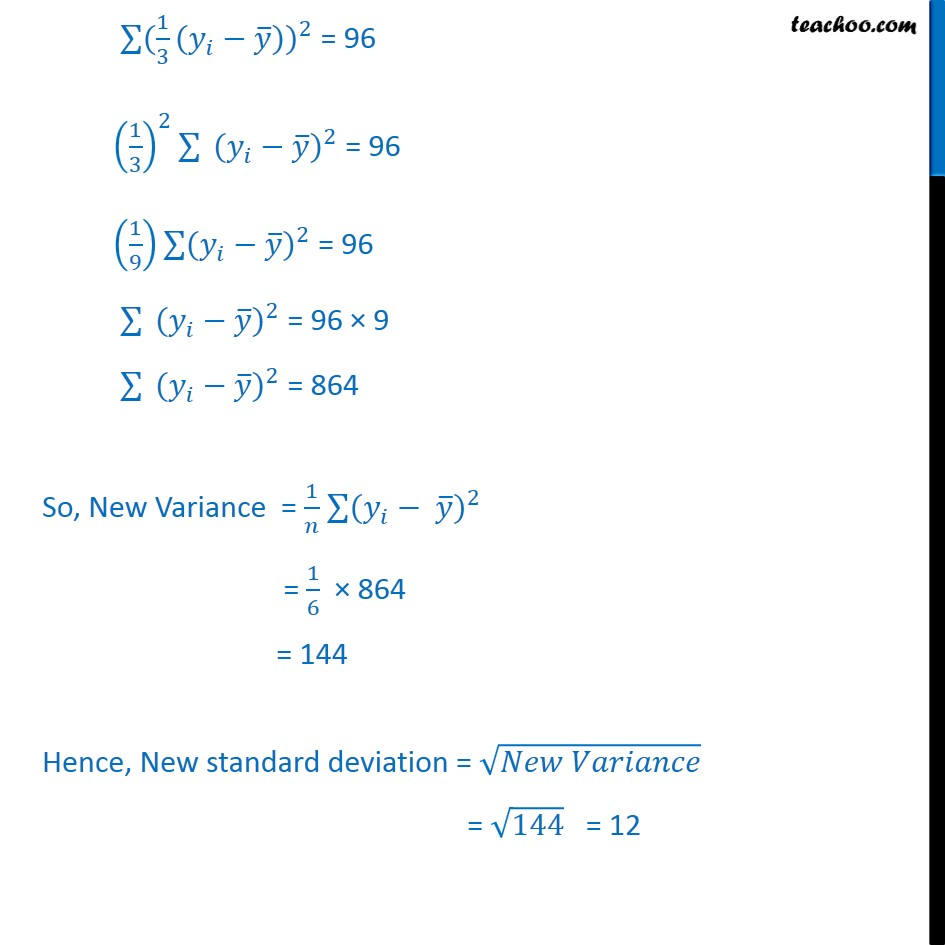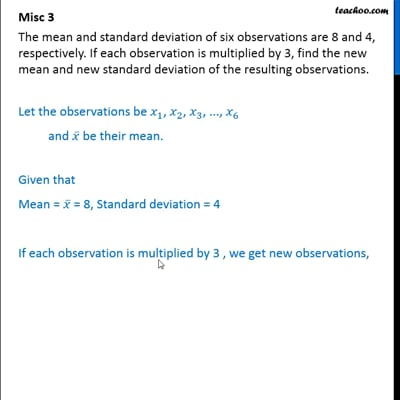This video is only available for Teachoo black users

Introducing your new favourite teacher - Teachoo Black, at only ₹83 per month

### Transcript

Misc 3 The mean and standard deviation of six observations are 8 and 4, respectively. If each observation is multiplied by 3, find the new mean and new standard deviation of the resulting observations. Let the observations be 𝑥﷮1﷯, 𝑥﷮2﷯, 𝑥﷮3﷯, ..., 𝑥﷮6﷯ and 𝑥﷯ be their mean. Given that Mean = 𝑥﷯ = 8, Standard deviation = 4 If each observation is multiplied by 3 , we get new observations, Let the new observations be 𝑦﷮1﷯, 𝑦﷮2﷯, 𝑦﷮3﷯, ..., 𝑦﷮6﷯ where 𝑦﷮𝑖﷯ = 3( 𝑥﷮𝑖﷯) Calculating new mean New mean = 1﷮𝑛﷯ ﷮﷮ 𝑦﷮𝑖﷯﷯ 𝑦﷯ = 1﷮6﷯ ﷮﷮ 3𝑥﷮𝑖﷯﷯ 𝑦﷯ = 3 × 1﷮6﷯ ﷮﷮ 𝑥﷮𝑖﷯﷯ 𝑦﷯ = 3 𝑥﷯ 𝑦﷯ = 3 × 8 𝑦﷯ = 24 So, New Mean = 24 Calculating new standard deviation First we find variance of the new observations i.e. New Variance = 1﷮n﷯ ﷮﷮( 𝑦﷮𝑖﷯﷯− 𝑦﷯)﷮2﷯ Given Old Standard deviation = 4 So, Old Variance = 42 = 16 Now, Old Variance = 1﷮𝑛﷯ ﷮﷮( 𝑥﷮𝑖﷯﷯− 𝑥﷯)﷮2﷯ 16 = 1﷮6﷯ ﷮﷮( 𝑥﷮𝑖﷯﷯− 𝑥﷯)﷮2﷯ 16 × 6 = ﷮﷮( 𝑥﷮𝑖﷯﷯− 𝑥﷯)﷮2﷯ 96 = ﷮﷮( 𝑥﷮𝑖﷯﷯− 𝑥﷯)﷮2﷯ ﷮﷮( 𝑥﷮𝑖﷯﷯− 𝑥﷯)﷮2﷯ = 96 ﷮﷮( 1﷮3﷯ 𝑦﷮𝑖﷯﷯− 1﷮3﷯ 𝑦﷯)﷮2﷯ = 96 ﷮﷮( 1﷮3﷯ (𝑦﷮𝑖﷯﷯− 𝑦﷯))﷮2﷯ = 96 1﷮3﷯﷯﷮2﷯ ﷮﷮ ( 𝑦﷮𝑖﷯﷯− 𝑦﷯)﷮2﷯ = 96 1﷮9﷯﷯ ﷮﷮( 𝑦﷮𝑖﷯﷯− 𝑦﷯)﷮2﷯ = 96 ﷮﷮ ( 𝑦﷮𝑖﷯﷯− 𝑦﷯)﷮2﷯ = 96 × 9 ﷮﷮ ( 𝑦﷮𝑖﷯﷯− 𝑦﷯)﷮2﷯ = 864 So, New Variance = 1﷮𝑛﷯ ﷮﷮( 𝑦﷮𝑖﷯﷯− 𝑦﷯)﷮2﷯ = 1﷮6﷯ × 864 = 144 Hence, New standard deviation = ﷮𝑁𝑒𝑤 𝑉𝑎𝑟𝑖𝑎𝑛𝑐𝑒﷯ = ﷮144﷯ = 12